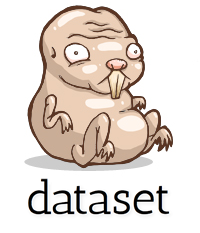## dataset: Lightweight ORM for development written in Pythondataset provides two key functions that make using SQL databases in
Python a breeze:

• A simple abstraction layer removes most direct SQL statements without the necessity for a full ORM model – essentially, databases can be used like a JSON file or NoSQL store.

• Database contents can be exported (frozen) using a sophisticated plain file generator with JSON and CSV support. Exports can be configured to include metadata and dynamic file names depending on the exported data. The exporter can also be used as a command-line tool, `datafreeze`.

## jOOQ: Full-featured, Java SQL-centric (non-ORM) database libraryGithub page: https://github.com/jOOQ/jOOQ

### Example 1:

``````create.selectFrom(BOOK)
.where(BOOK.PUBLISHED_IN.eq(2011))
.orderBy(BOOK.TITLE)
``````

### Example 2:

``````create.select(AUTHOR.FIRST_NAME, AUTHOR.LAST_NAME, count())
.from(AUTHOR)
.join(BOOK).on(AUTHOR.ID.equal(BOOK.AUTHOR_ID))
.where(BOOK.LANGUAGE.eq("DE"))
.and(BOOK.PUBLISHED.gt(date("2008-01-01")))
.groupBy(AUTHOR.FIRST_NAME, AUTHOR.LAST_NAME)
.having(count().gt(5))
.orderBy(AUTHOR.LAST_NAME.asc().nullsFirst())
.limit(2)
.offset(1)
``````

### Type-safety example:

``````select().from(t).where(t.a.eq(select(t2.x).from(t2));
// Type-check here: ---------------> ^^^^
select().from(t).where(t.a.eq(any(select(t2.x).from(t2)));
// Type-check here: -------------------> ^^^^
select().from(t).where(t.a.in(select(t2.x).from(t2));
// Type-check here: ---------------> ^^^^
``````